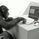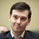‎2380‎ مشاهدة
"All stocks in the market have unique personalities in terms of implied volatility (their option prices). For example, one stock might have an implied volatility of 30%, while another has an implied volatility of 50%. Even more, the 30% IV stock might usually trade with 20% IV, in which case 30% is high. On the other hand, the 50% IV stock might usually trade with 75% IV, in which case 50% is low.

So, how do we determine whether a stock's option prices (IV) are relatively high or low?

The solution is to compare each stock's IV against its historical IV levels. We can accomplish this by converting a stock's current IV into a rank or percentile.

Implied Volatility Rank (IV Rank) Explained
Implied volatility rank (IV rank) compares a stock's current IV to its IV range over a certain time period (typically one year).

Here's the formula for one-year IV rank:

(Current IV - 1 Year Low IV) / (1 Year High IV - 1 Year Low IV) * 100

For example, the IV rank for a 20% IV stock with a one-year IV range between 15% and 35% would be:

(20% - 15%) / (35% - 15%) = 25%

An IV rank of 25% means that the difference between the current IV and the low IV is only 25% of the entire IV range over the past year, which means the current IV is closer to the low end of historical levels of implied volatility .

Furthermore, an IV rank of 0% indicates that the current IV is the very bottom of the one-year range, and an IV rank of 100% indicates that the current IV is at the top of the one-year range.

Implied Volatility Percentile (IV Percentile) Explained
Implied volatility percentile (IV percentile) tells you the percentage of days in the past that a stock's IV was lower than its current IV.

Here's the formula for calculating a one-year IV percentile:

Number of trading days below current IV / 252 * 100

As an example, let's say a stock's current IV is 35%, and in 180 of the past 252 days, the stock's IV has been below 35%. In this case, the stock's 35% implied volatility represents an IV percentile equal to:

180/252 * 100 = 71.42%

An IV percentile of 71.42% tells us that the stock's IV has been below 35% approximately 71% of the time over the past year.

Applications of IV Rank and IV Percentile
Why does it help to know whether a stock's current implied volatility is relatively high or low? Well, many traders use IV rank or IV percentile as a way to determine appropriate strategies for that stock.

For example, if a stock's IV rank is 90%, then a trader might look to implement strategies that profit from a decrease in the stock's implied volatility , as the IV rank of 90% indicates that the stock's current IV is at the top of its range over the past year (for a one-year IV rank).

On the other hand, if a stock's IV rank is 0%, then traders might look to implement strategies that profit from an increase in implied volatility , as the IV rank of 0% indicates the stock's current implied volatility is at the bottom of its range over the past year."

This script approximates IV by using the VIX products, which calculate the 30-day implied volatility of the specified security.

*Includes an option for repainting -- default value is true, meaning the script will repaint the current bar.
False = Not Repainting = Value for the current bar is not repainted, but all past values are offset by 1 bar.
True = Repainting = Value for the current bar is repainted, but all past values are correct and not offset by 1 bar.
In both cases, all of the historical values are correct, it is just a matter of whether you prefer the current bar to be realistically painted and the historical bars offset by 1, or the current bar to be repainted and the historical data to match their respective price bars.
As explained by TradingView,`f_security()` is for coders who want to offer their users a repainting/no-repainting version of the HTF data.
ملاحظات الأخبار: Added more markets from the VIX family.
ملاحظات الأخبار: Added the Interest Rate Swap Volatility Index and the Crude Oil Volatility Index.
ملاحظات الأخبار: Added the MOVE Index. The Merrill Lynch Option Volatility Estimate (MOVE) Index is a yield curve weighted index of the normalized implied volatility on 1-month Treasury options which are weighted on the 2, 5, 10, and 30 year contracts.
نص برمجي مفتوح المصدر

قام مؤلف هذا النص البرمجي بنشره وجعله مفتوح المصدر، بحيث يمكن للمتداولين فهمه والتحقق منه، وهو الأمر الذي يدخل ضمن قيم TradingView. تحياتنا للمؤلف! يمكنك استخدامه مجانًا، ولكن إعادة استخدام هذا الكود في منشور تحكمه قواعد الموقع. يمكنك جعله مفضلاً لاستخدامه على الرسم البياني.

هل تريد استخدام هذا النص البرمجي على الرسم البياني؟

## التعليقاتThanks G, I hope the wifi is treating you nicely in the boonies
ردFarmer_George
@Farmer_George, IV rank can go below 0 and above 100, IV percentile cannot. Look up the article "HOW CAN IV RANK BE OVER 100 OR LESS THAN 0?" by Tastyworks to see why.
ردFarmer_George
@Farmer_George, Good question. From the website spindices: "The VIX measures the implied volatility of the S&P 500 (SPX) for the next 30 days ... VIX measures implied volatility by averaging the weighted prices of a wide range of put and call options. When investors buy and sell options, the positions they take—either puts or calls—the prices they are willing to pay, and the strike prices they choose, all reflect how much and how quickly they think the underlying index level will move. In fact, that’s what volatility is: the pace and amount of change. VIX uses options prices rather than stock prices in its calculation because options prices reflect the volatility buyers and sellers expect. That’s what implied in implied volatility means."

The underlying model for options prices is in fact Black-Scholes, and VIX indices are calculated based on options prices.

While VIX measures 30 day IV, VIX9D measures 9 day IV, VIX3M measures 3 month IV, and so on.
رد